## 3.2.3 LS Surface Vector Motion

The level set is used to describe the motion of a surface when its rate of growth is given in the surface normal direction. However, when a LS surface fully encompases a material in all directions, a rate of growth in the normal direction would result in a volume expansion of the material. When the entire material must be moved in a single direction, independent of the surface normals, while preserving the material's surface normals, curature, and volume, the LS surface must be advanced using the velocity equation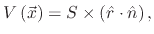(92)

where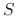is the speed of the surface motion,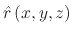is the unit vector in the direction of the desired motion, and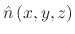is the unit normal vector to the surface. Equation (3.21) is applied to a sphere moving towards a flat disk in Figure 3.6. The simulation depicts a sphere with a diameter of 100 grid units moving downward at a speed of 1 grid unit per second, towards a stationary surface used as a reference point. The total translation is 200 grid units (50 grid units per image) prior to contact with the bottom surface. The sphere does not appear to experience any deformation.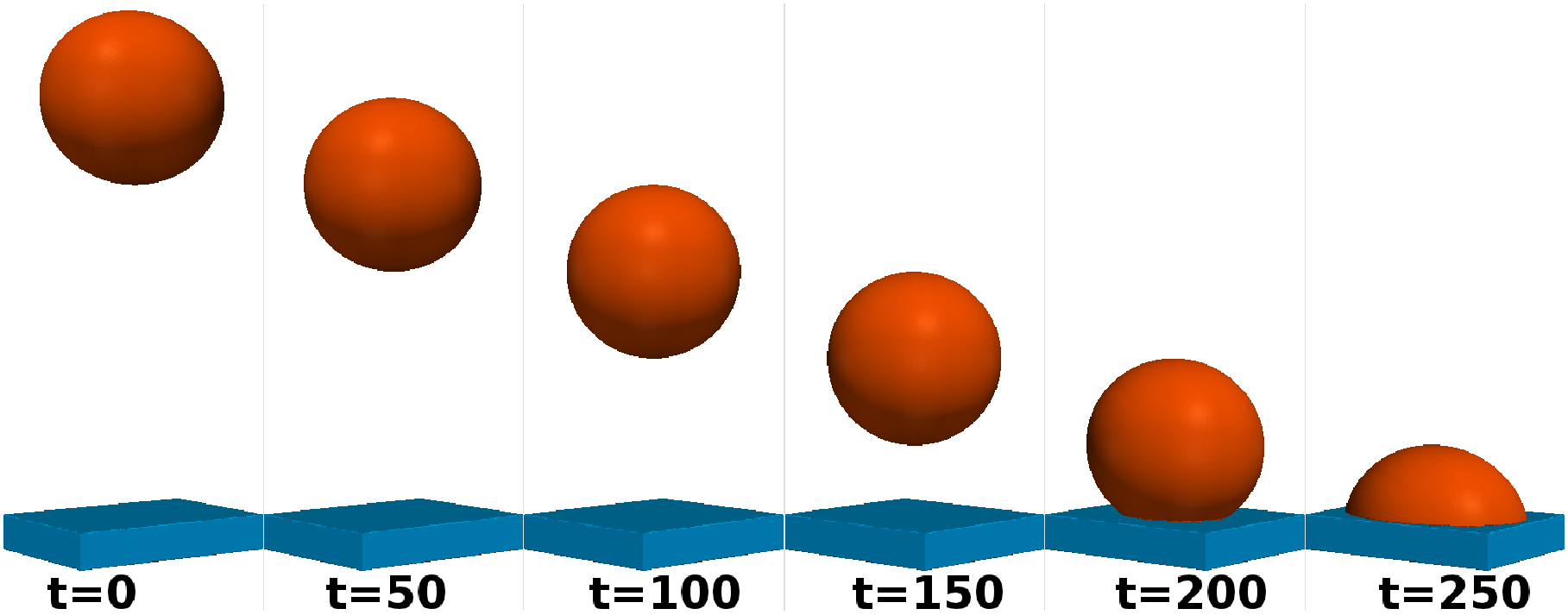The type of simulation depicted in Figure 3.6 is quite sensitive to errors because any error in the surface definition after a single time step will multiply with subsequent steps. The grid also plays a role in the accuracy of the generated result. A finer grid will result in a more accurate representation of the initial surface after it has transposed. Therefore, depending on the accuracy required, the simulation can require extensive amounts of memory, processing power, and time.

The grid density will determine how well the surface curvature will be transfered as it moves through the grid. It is not recommended to perform simulations where the surface will transpose a significant distance away from its original location as it is inevitable that many surface edges will be lost. However, for slight movements of a surface, such as the bending that a nitride mask experiences during oxidation, this type of LS motion can be implemented.

L. Filipovic: Topography Simulation of Novel Processing Techniques